###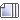Author Topic: Seven Ineffective Coding Habits of Many Programmers  (Read 5550 times)

####Hooman

• Hero Member
•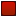•• Posts: 4873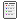##### Re: Seven Ineffective Coding Habits of Many Programmers
« Reply #25 on: October 14, 2018, 12:49:03 PM »
Hmm, I was reading something recently about mixing levels of abstraction, and how that can lead to hard to read code. Some of the examples involved if statement expressions.

In terms of adding comments to label if/else if branches, I think that would mostly be necessary if you were mixing levels of abstraction. One solution might be to extract the checks into a method, where the name of the method provides a higher level of abstraction, and hence labelling of the if/else if branches.

The main difference between pointers and references are that references are non-nullable and constant. It's a compile error if you try to assign null to a reference. Instead of documenting that a pointer can not accept null, consider using a reference instead. A reference would imply the value needs to point to something valid.

Indeed, you have to go out of your way to trick the compiler into getting a null value stored in a reference variable. One way is to first create a pointer, set it to null, and then create a reference that points to the same thing as the pointer. Failing that, you'd need an ugly cast, or a really awful union, to subvert the type system.
Code: cpp [Select]
``Obj& ref = 0;  // Error!Obj* ptr = 0;Obj& ref = *ptr; // Okay! (Does not check if ptr is null)Obj& ref = *(Obj*)0;  // Okay! (Casting has subverted the type checks)union {  Obj* ptr;  // References can not be stored directly in a union, so wrap in a struct  struct Ref {    // Explicitly define a constructor, since otherwise it is deleted due to the reference    Ref();    Obj& ref;  };} unionVar;unionVar.ptr = 0;  // Okay!  (unionVar.ref is now also null)``

Another indication you might want to use a reference, is if the pointer itself is constant. References are only ever set when they are defined. They can not be re-assigned. This corresponds to a const pointer (as opposed to a pointer to a const).

Or, put the other way, the two reasons for using a pointer rather than a reference, is to support using null values, or to be able to re-assign the pointer dynamically. If you don't need either feature, I'd recommend using a reference over using a pointer.

It's probably a good idea to use division rather than shift when the actual intent really is to divide. Although easy to forget due to it's prevalence, the shift is an optimization, and one that isn't clear to non-programmers, or people unfamiliar with binary numbers. Using a division really is more clear. Plus, I don't know of any compiler that can't optimize a division by a constant power of 2 into a bit shift.

There is however one gotcha. The rounding mode (towards 0) for signed integers does not match up with a simple bit shift. If you try to divide by a constant power of 2, and only care about positive numbers, but forget to declare the variable as unsigned, you'll get a less efficient opcode sequence with extra instructions to adjust for the rounding mode.

Code: cpp [Select]
``int divideBy2(int value) {  return value / 2;}``

Code: asm [Select]
``_Z9divideBy2i:.LFB1564:	.cfi_startproc	mov	eax, edi  ; Copy the value  (we need some scratch space to work with the sign bit)	shr	eax, 31   ; Shift right (logical), moving the sign bit to lowest bit position	add	eax, edi  ; Add 1 (round up towards 0) if value was negative, otherwise add 0 (positive)	sar	eax       ; Shift right (arithmetic), divide by 2, maintain signdness of result	ret               ; Return result (in eax register)	.cfi_endproc``

For unsigned values, a rounding mode of towards 0 matches a rounding mode of round down, which is a simple bit shift.
Code: cpp [Select]
``unsigned int divideBy2(unsigned int value) {  return value / 2;}``

Code: asm [Select]
``_Z9divideBy2j:.LFB1565:	.cfi_startproc	mov	eax, edi  ; Copy the value  (this could be removed if the method was inlined)	shr	eax       ; Shift right (logical): Unsigned divide by 2	ret               ; Return result (in eax register)	.cfi_endproc``

####Arklon

• Hero Member
••• Posts: 1226##### Re: Seven Ineffective Coding Habits of Many Programmers
« Reply #26 on: October 14, 2018, 12:57:09 PM »
(e.g., /* nullptr is never passed so it's not checked for here */)
Invariants like that should probably be asserts, which are self-documenting anyway.

####Hooman

• Hero Member
••• Posts: 4873##### Re: Seven Ineffective Coding Habits of Many Programmers
« Reply #27 on: October 14, 2018, 01:11:00 PM »
That's also a very valid point. I don't usually think to use asserts. They're also more widely applicable to a wide array of things you might want to check for, not just that a pointer isn't null.

I suppose one reason I don't often use asserts is they only work in debug mode, and I used to always just build and test in release mode. Made sense to me to be testing what was going to be shipped.

In hindsight, not using debug builds meant my skills with certain debugging tools was a bit lacking. On a few occasions I found myself debugging the output assembly code in OllyDbg, rather than source code in the MSVC debugger, just because I was more comfortable with OllyDbg. I kind of had to roll my eyes at myself for that though.

In this particular case, I think I would still prefer references, since you get some minimal compile time checks regardless of the build settings, and there is still no runtime overhead.

####Arklon

• Hero Member
••• Posts: 1226##### Re: Seven Ineffective Coding Habits of Many Programmers
« Reply #28 on: October 14, 2018, 01:15:00 PM »
Another indication you might want to use a reference, is if the pointer itself is constant. References are only ever set when they are defined. They can not be re-assigned. This corresponds to a const pointer (as opposed to a pointer to a const).

Or, put the other way, the two reasons for using a pointer rather than a reference, is to support using null values, or to be able to re-assign the pointer dynamically. If you don't need either feature, I'd recommend using a reference over using a pointer.
A lot of projects' coding standards only allow const references for function arg types (with the exception of r-value references), though, and if it needs to be mutable then it needs to be a pointer. That means you have to put e.g. "function(..., &variableToModify)" in calls to such functions, which helps distinguish output args from inputs in the code.

Quote
It's probably a good idea to use division rather than shift when the actual intent really is to divide. Although easy to forget due to it's prevalence, the shift is an optimization, and one that isn't clear to non-programmers, or people unfamiliar with binary numbers. Using a division really is more clear. Plus, I don't know of any compiler that can't optimize a division by a constant power of 2 into a bit shift.

There is however one gotcha. The rounding mode (towards 0) for signed integers does not match up with a simple bit shift. If you try to divide by a constant power of 2, and only care about positive numbers, but forget to declare the variable as unsigned, you'll get a less efficient opcode sequence with extra instructions to adjust for the rounding mode.
The real gotcha to this is that the compiler can only optimize div/mod pow2 for compile-time constants. If you're writing code that divides by a non-constexpr variable, where you assume it must be a power of 2, then to optimize that you have to explicitly write out the bit twiddling yourself and not use / or %.
« Last Edit: October 14, 2018, 01:18:07 PM by Arklon »

####Hooman

• Hero Member
••• Posts: 4873##### Re: Seven Ineffective Coding Habits of Many Programmers
« Reply #29 on: October 14, 2018, 01:42:04 PM »
That's a good point about coding standards prohibiting it. I've read a number of times of complains about how references parameters obscure the notion of a pointer being used at the call site, since the address taking is done automatically without syntactic markup at the point of call. I suppose I've never been much bothered by it myself, though do admit that's a fairly valid point.

I guess I've generally found that with most APIs I've used, it's pretty obvious when a parameter is mutable, or an out parameter. It's usually obviously built into the name and purpose of the function. Though that won't be true of code in general. There's nothing stopping you from being sneaky or obscure about what you modify, or explaining something badly.

Ok, so pointers that can not be null, are const, and point to const (or are not restricted by code guidelines), should probably be references.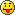Can you give an example of a non-constexpr variable where the compiler can not optimize a division into a bit shift, but the programmer could explicitly code it?

If the divisor was an actual variable value, I assume it would be faster to just do the divide, then to try and convert it to a shift value and then do a bit shift. I remember seeing bit twiddling hacks to convert from powers of 2 to the bit number, and I don't remember it being all that simple.

Or perhaps there's a simple example where the compiler fails to optimize for something that really is const, but wasn't deemed constexpr?

####Arklon

• Hero Member
••• Posts: 1226##### Re: Seven Ineffective Coding Habits of Many Programmers
« Reply #30 on: October 14, 2018, 02:12:40 PM »
Can you give an example of a non-constexpr variable where the compiler can not optimize a division into a bit shift, but the programmer could explicitly code it?

If the divisor was an actual variable value, I assume it would be faster to just do the divide, then to try and convert it to a shift value and then do a bit shift. I remember seeing bit twiddling hacks to convert from powers of 2 to the bit number, and I don't remember it being all that simple.
You don't. You have to work with log2 numbers. This might involve having to do bit twiddling once which itself is more expensive than a div - one way to do it is, basically, take (pow2 number) - 1, and then either use the Hamming weight algorithm or the SSE4 popcnt intrinsic (both are constant time complexity), or if we're using intrinsics maybe just bitscan forward would be fastest. However, when you are going to do many divisions by the same pow2 number, it can end up being cheaper to do the log2 conversion once so you can do bit shifts thereafter, rather than doing div every time.

The mod case is trivial, you just replace (A % B) with (A & (B - 1)).

Quote
Or perhaps there's a simple example where the compiler fails to optimize for something that really is const, but wasn't deemed constexpr?
This sort of thing can happen with function inlining. You call a non-constexpr function with a constexpr arg, which is used as the divisor or whatever else, so then the arg becomes non-constexpr. But then, the compiler inlines it, and sadly compilers aren't very good about recognizing this sort of situation and doesn't go back and realize it could treat that arg as a constexpr now.
« Last Edit: October 14, 2018, 09:51:44 PM by Arklon »

####Hooman

• Hero Member
••• Posts: 4873##### Re: Seven Ineffective Coding Habits of Many Programmers
« Reply #31 on: October 15, 2018, 05:49:30 AM »
Ahh, that makes sense. Frontload the work. I was stuck thinking of a single divide rather than an array divide.

Thanks for the pop count link, that was good review. The bit manipulations has a rather interesting structure.

As for the algorithm complexity, if you were being pedantic, any algorithm is constant time when you fix the input size. As the pop count instruction works on a fixed number of bits (the register size), it is of course constant time. Though you may notice from the algorithm, the actual number of instructions depends on the number of bits involved in the counting. Looks to be logarithmic in the number of bits, which is pretty efficient. And of course, having a machine opcode to do it is always handy. Looks like it can be done quite efficiently at the hardware level.

I do rather enjoy the conversion (A % B) => (A & (B - 1)) when B is a power of 2. I use a slight variant of that for rounding up to the next multiple of a power of 2: roundUp(value, multiple) = (value + (multiple - 1)) & ~(multiple - 1)

I tried your suggestion to pass a constexpr through a non-constexpr function. It seems the g++ optimizer is too good. I was not able to fool it with my simple test:

Code: cpp [Select]
``unsigned int addOne(unsigned int x) {  return x + 1;}unsigned int divideByTwo(unsigned int x) {  return x / addOne(1);}``

The result of g++ -c -S -masm=intel -O2 constExprArg.cpp is:
Code: asm [Select]
``_Z6addOnej:.LFB0:	.cfi_startproc	lea	eax, 1[rdi]	ret	.cfi_endproc..._Z11divideByTwoj:.LFB1:	.cfi_startproc	mov	eax, edi	shr	eax         ; Shift right (logical): Divide by 2	ret	.cfi_endproc``

####Arklon

• Hero Member
••• Posts: 1226##### Re: Seven Ineffective Coding Habits of Many Programmers
« Reply #32 on: October 15, 2018, 10:27:51 AM »
Quote
As for the algorithm complexity, if you were being pedantic, any algorithm is constant time when you fix the input size. As the pop count instruction works on a fixed number of bits (the register size), it is of course constant time. Though you may notice from the algorithm, the actual number of instructions depends on the number of bits involved in the counting. Looks to be logarithmic in the number of bits, which is pretty efficient. And of course, having a machine opcode to do it is always handy. Looks like it can be done quite efficiently at the hardware level.
Not exactly, actually. Whether you're dealing with 8, 16, 32, or 64 bits, (whatever the native bit depth of the system is) the algorithm is almost identical, the only differences being the 0x5555/0x3333/etc. constants would be truncated to whatever bit depth you're interested in, and the last bit shift in the algorithm is ">> (bitDepth - 8 )".

Quote
I tried your suggestion to pass a constexpr through a non-constexpr function. It seems the g++ optimizer is too good. I was not able to fool it with my simple test:
Yeah, sometimes the compiler is able to optimize it away, but it's not totally reliable, and YMMV depending on the compiler. Math operations are probably the best case for this though, whereas more special-case things like memcpy/memset inlining when using a constexpr size tends to not work properly with multiple degrees of inlining.
« Last Edit: October 15, 2018, 06:48:55 PM by Arklon »

####Hooman

• Hero Member
••• Posts: 4873##### Re: Seven Ineffective Coding Habits of Many Programmers
« Reply #33 on: October 17, 2018, 08:20:38 PM »
You're right about truncating the constants, though I also believe you'll need to adjust the number of constants based on the bit size. From the Wikipedia article you linked on Hamming Weight:
Quote
For processors lacking those features, the best solutions known are based on adding counts in a tree pattern.

The height of that tree depends on the number of bits at the bottom.

If you were to look at the first algorithm listed:
Code: cpp [Select]
``//types and constants used in the functions below//uint64_t is an unsigned 64-bit integer variable type (defined in C99 version of C language)const uint64_t m1  = 0x5555555555555555; //binary: 0101...const uint64_t m2  = 0x3333333333333333; //binary: 00110011..const uint64_t m4  = 0x0f0f0f0f0f0f0f0f; //binary:  4 zeros,  4 ones ...const uint64_t m8  = 0x00ff00ff00ff00ff; //binary:  8 zeros,  8 ones ...const uint64_t m16 = 0x0000ffff0000ffff; //binary: 16 zeros, 16 ones ...const uint64_t m32 = 0x00000000ffffffff; //binary: 32 zeros, 32 onesconst uint64_t h01 = 0x0101010101010101; //the sum of 256 to the power of 0,1,2,3...//This is a naive implementation, shown for comparison,//and to help in understanding the better functions.//This algorithm uses 24 arithmetic operations (shift, add, and).int popcount64a(uint64_t x){    x = (x & m1 ) + ((x >>  1) & m1 ); //put count of each  2 bits into those  2 bits     x = (x & m2 ) + ((x >>  2) & m2 ); //put count of each  4 bits into those  4 bits     x = (x & m4 ) + ((x >>  4) & m4 ); //put count of each  8 bits into those  8 bits     x = (x & m8 ) + ((x >>  8) & m8 ); //put count of each 16 bits into those 16 bits     x = (x & m16) + ((x >> 16) & m16); //put count of each 32 bits into those 32 bits     x = (x & m32) + ((x >> 32) & m32); //put count of each 64 bits into those 64 bits     return x;}``

The constant m32 would by useless as a bitmask on 32-bit operations, as would the x >> 32 operation. Accounting for the halving/truncating of the constants, and removal of dead operations, the 32-bit equivalent should be:
Code: cpp [Select]
``const uint32_t m1  = 0x55555555; //binary: 0101...const uint32_t m2  = 0x33333333; //binary: 00110011..const uint32_t m4  = 0x0f0f0f0f; //binary:  4 zeros,  4 ones ...const uint32_t m8  = 0x00ff00ff; //binary:  8 zeros,  8 ones ...const uint32_t m16 = 0x0000ffff; //binary: 16 zeros, 16 ones ...int popcount32a(uint32_t x){    x = (x & m1 ) + ((x >>  1) & m1 ); //put count of each  2 bits into those  2 bits     x = (x & m2 ) + ((x >>  2) & m2 ); //put count of each  4 bits into those  4 bits     x = (x & m4 ) + ((x >>  4) & m4 ); //put count of each  8 bits into those  8 bits     x = (x & m8 ) + ((x >>  8) & m8 ); //put count of each 16 bits into those 16 bits     x = (x & m16) + ((x >> 16) & m16); //put count of each 32 bits into those 32 bits     return x;}``

That is, one less tree operation for half the number of bits. Or one extra tree operation if you double the number of bits.

Well, I think I got wildly off topic on this thread####Arklon

• Hero Member
••• Posts: 1226##### Re: Seven Ineffective Coding Habits of Many Programmers
« Reply #34 on: October 17, 2018, 09:51:40 PM »
That's not the most optimized form of the algorithm. Here:
Code: [Select]
``constexpr uint64 m1 = 0x5555555555555555;constexpr uint64 m2 = 0x3333333333333333;constexpr uint64 m3 = 0x0F0F0F0F0F0F0F0F;constexpr uint64 m4 = 0x0101010101010101;uint64 PopCount64(uint64 x) {  x = x - ((x >> 1) & m1);  x = (x & m2) + ((x >> 2) & m2);  x = (((x + (x >> 4)) & m3) * m4) >> ((64 /* bit depth */) - 8);  return x;}``
« Last Edit: October 17, 2018, 09:53:37 PM by Arklon »

####Hooman

• Hero Member
••• Posts: 4873##### Re: Seven Ineffective Coding Habits of Many Programmers
« Reply #35 on: October 18, 2018, 03:33:25 AM »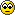Well, if you want to get pedantic...The point was easier to illustrate with the first version. The second version is basically identical, but strays from the pattern by omitting a few operations that aren't actually needed due to range restrictions. It is asymptotically identical.

The third version is an optimization of the second algorithm, that further modifies the later part. It hides some of the complexity in a multiplication operation. The note about about "...on machines with fast multiplication" is particularly relevant. However, the asymptotic complexity of the multiplication is actually greater than the base algorithm, so this implementation actually scales less well with bit size. It's only really an optimization for small bit sizes, where you have a fast hardware multiply instruction, but no fast hardware bitcount instruction.

See: Computational Complexity of Mathematical Operations

There are multiple multiplication algorithms listed, each with their complexity, and all of them are super linear. For large bit sizes, this would dominate, making this algorithm less optimal.

Since I omitted it previously, technically you should also consider the bit-complexity of the additions used in the earlier algorithms. The computational complexity of each addition is linear for schoolbook addition with ripple carry. You can get faster addition with a carry lookahead generator though, such as in the Carry-Lookahead Adder. That reduces the bit complexity of the addition to log(n) in the number of bits. Further, the additions do not use the full bit size of the input registers, so saying log(n), where n is the register size is actually too big. As there is a constant number of additions per level of the computation tree, that comes to at most (log(n))^2 complexity. So in short, still sub-linear.

But again, if you fix the bit size, it all comes out as constant time in the end.